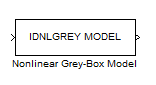# Nonlinear Grey-Box Model

Simulate nonlinear grey-box model in Simulink software

## Library

System Identification Toolbox

•## Description

Simulates systems of nonlinear grey-box (`idnlgrey`) models.

### Input

Input signal to the model.

### Output

Output signal from the model.

## Parameters

IDNLGREY model

Name of `idnlgrey` variable in the MATLAB® workspace.

Initial state

Specify the initial states as one of the following:

• `'z'`: Specifies zero, which corresponds to a system starting from rest.

• `'m'`: Specifies the internal initial states of the model.

• Vector of size equal to the number of states in the `idnlgrey` object.

• An initial state structure array. For information about creating this structure, type `help idnlgrey/sim` in the MATLAB Command Window.

## Version History

Introduced in R2008a